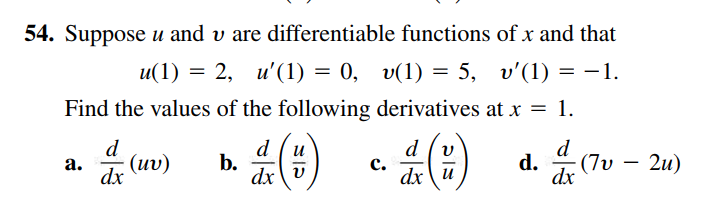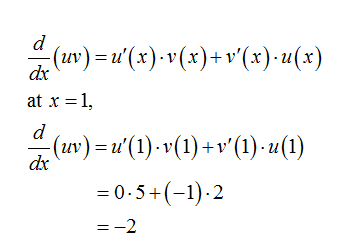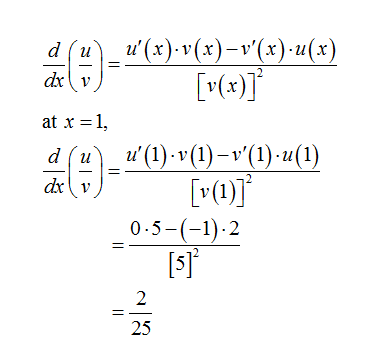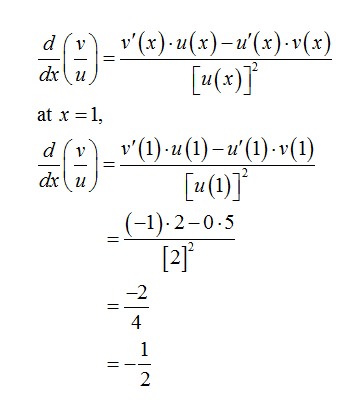# 54. Suppose u and v are differentiable functions of x and thatu(1) = 2, u'(1) = 0, v(1) = 5, v'(1) = –1.Find the values of the following derivatives at x = 1.dd., (7υ-2u)b.dx v(uv)a.c.dx\udxdx

Question
4 views

Can i get help with this problem step by step please?help_outlineImage Transcriptionclose54. Suppose u and v are differentiable functions of x and that u(1) = 2, u'(1) = 0, v(1) = 5, v'(1) = –1. Find the values of the following derivatives at x = 1. d d. , (7υ-2u) b. dx v (uv) a. c. dx\u dx dx fullscreen
check_circle

a). Differentiating by using product rule, we getb). Differentiating by using quotient rule, we getc). Differentiating by using quotient rule, we get...

### Want to see the full answer?

See Solution

#### Want to see this answer and more?

Solutions are written by subject experts who are available 24/7. Questions are typically answered within 1 hour.*

See Solution
*Response times may vary by subject and question.
Tagged in